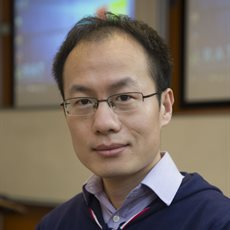# Dr Yuzhao Wang BSc PhDSchool of Mathematics
Associate Professor

## Contact details

School of Mathematics
Watson Building
University of Birmingham
Edgbaston
Birmingham
B15 2TT
UK

Yuzhao Wang is an Associate Professor in the School of Mathematics.

Yuzhao’s primary research area is mathematical analysis of nonlinear dispersive partial differential equations (PDEs), with tools from harmonic analysis, probability theory, and dynamical systems.

Personal webpage.

## Qualifications

• PhD in Mathematics, Peking University, 2010
• BSc in Mathematics, Jilin University, 2005

## Biography

Yuzhao Wang has joined University of Birmingham since 2017 as a Lecture. He was promoted to Senior Lecture in 2021. Previously Yuzhao held research positions at the University of Edinburgh and Memorial University.

LC Real Analysis

## Research

Nonlinear Partial Differential Equations and Harmonic Analysis. In particular, the study of nonlinear dispersive PDEs such as nonlinear Schrödinger equations, nonlinear wave equations, and the KdV equation by using techniques from PDEs, Harmonic Analysis, and Probability theory.

Mainly, well-posedness (existence, uniqueness, and stability of solutions) in both deterministic and probabilistic settings, existence of invariant measures, Strichartz estimates in different settings, etc. Also, interested in Fourier restriction theory and decoupling theory.

## Publications

### Recent publications

#### Article

Liang, R & Wang, Y 2022, 'Gibbs measure for the focusing fractional NLS on the torus', SIAM Journal on Mathematical Analysis, vol. 54, no. 6, pp. 6096-6118. https://doi.org/10.1137/21M1445946

Oh, T, Wang, Y & Zine, Y 2022, 'Three-dimensional stochastic cubic nonlinear wave equation with almost space-time white noise', Stochastics and Partial Differential Equations: Analysis and Computations, vol. 10, no. 3, pp. 898-963. https://doi.org/10.1007/s40072-022-00237-x

Oh, T, Robert, T, Sosoe, P & Wang, Y 2021, 'Invariant Gibbs dynamics for the dynamical sine-Gordon model', Proceedings of the Royal Society of Edinburgh Section A: Mathematics, vol. 151, no. 5, pp. 1450-1466. https://doi.org/10.1017/prm.2020.68

Oh, T & Wang, Y 2021, 'Normal form approach to the one-dimensional periodic cubic nonlinear Schrödinger equation in almost critical Fourier-Lebesgue spaces', Journal d'Analyse Mathématique, vol. 143, no. 2, pp. 723-762. https://doi.org/10.1007/s11854-021-0168-1

Oh, T & Wang, Y 2021, 'On global well-posedness of the modified KdV equation in modulation spaces', Discrete and Continuous Dynamical Systems- Series A, vol. 41, no. 6, pp. 2971-2992. https://doi.org/10.3934/dcds.2020393

Oh, T, Robert, T & Wang, Y 2021, 'On the Parabolic and Hyperbolic Liouville Equations', Communications in Mathematical Physics, vol. 387, no. 3, pp. 1281-1351. https://doi.org/10.1007/s00220-021-04125-8

Oh, T, Robert, T, Sosoe, P & Wang, Y 2021, 'On the two-dimensional hyperbolic stochastic sine-Gordon equation', Stochastics and Partial Differential Equations: Analysis and Computations, vol. 9, no. 1, pp. 1–32. https://doi.org/10.1007/s40072-020-00165-8

Oh, T & Wang, Y 2020, 'Global well-posedness of the one-dimensional cubic nonlinear Schrödinger equation in almost critical spaces', Journal of Differential Equations, vol. 269, no. 1, pp. 1-29. https://doi.org/10.1016/j.jde.2019.12.017

Oh, T, Pocovnicu, O & Wang, Y 2020, 'On the stochastic nonlinear Schrödinger equations with nonsmooth additive noise', Kyoto Journal of Mathematics, vol. 60, no. 4, pp. 1227-1243. https://doi.org/10.1215/21562261-2019-0060

Oh, T, Tzvetkov, N & Wang, Y 2020, 'Solving the 4NLS with white noise initial data', Forum of Mathematics, Sigma. https://doi.org/10.1017/fms.2020.51

Forlano, J, Oh, T & Wang, Y 2020, 'Stochastic nonlinear Schrödinger equation with almost space-time white noise', Journal of the Australian Mathematical Society, vol. 109, no. 1, pp. 44-67. https://doi.org/10.1017/S1446788719000156

Wang, W & Wang, Y 2019, 'Liouville-type theorems for the stationary MHD equations in 2D', Nonlinearity, vol. 32, no. 11, pp. 4483-4505. https://doi.org/10.1088/1361-6544/ab32a6

Oh, T, Pocovnicu, O & Wang, Y 2019, 'On the stochastic nonlinear Schrödinger equations with non-smooth additive noise', Kyoto Journal of Mathematics.

Pocovnicu, O & Wang, Y 2018, 'An Lp-theory for almost sure local well-posedness of the nonlinear Schrödinger equations', Comptes Rendus Mathematique, vol. 356, no. 6, pp. 637-643. https://doi.org/10.1016/j.crma.2018.04.009

Oh, T & Wang, Y 2018, 'Global well-posedness of the periodic cubic fourth order NLS in negative Sobolev spaces', Forum of Mathematics, Sigma, vol. 6, e5. https://doi.org/10.1017/fms.2018.4